### Numerical Methods–Unit 1 Two Marks with Answers

Anna University

NUMERICAL METHODS

UNIT-I

SOLUTION OF EQUATIONS AND EIGENVALUE PROBLEMS

Two Marks with Answes

1. If a function f(x) = 0 is continuous in the interval (a, b) and if f (a) and f (b) are of opposite signs. Then one of the root lies between a and b.

2. Example of Algebraic equation:

(i) x3 – 2x + 5 = 0;

(ii) 2x3 – 3x – 6 = 0.

3. Example of Transcendental equation:

(i) x – cosx = 0;

(ii) xex -2 = 0;

4. Regula Falsi Method: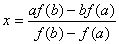(First iteration of Regula Falsi Method).

5. Iterative Method: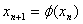.

6. Convergence condition of iterative method is

7. Order of convergence of iterative method is linear (i.e.) 1.

8. Newton Raphson’s Method (Method of Tangents):

9. Convergence condition of N-R method is

10. Order of convergence of Newton’s method is quadratic (i.e.) 2.

11. Direct Method:

(i) Gauss Elimination Method,

(ii) Gauss Jordan Method.

12. Indirect Method (or) Iteration Method:

(i) Gauss-Jacobi Method,

(ii) Gauss-Seidel Method.

13. Gauss Elimination Method: To reduce the augmented matrix [A, B] to upper triangular matrix. Then, using Back Substitution method we’ve to find the unknowns.

14. Gauss Jordan Method: To reduce the augmented matrix [A, B] to diagonal matrix. Finally this system of equation each has only one unknown and then we’ve to find the unknowns directly.

15. Diagonally Dominant: An n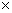n matrix A is said to be diagonally dominant if the absolute value of each leading diagonal element is greater than or equal to the sum of the absolute values of the remaining elements in that row.

Given system of equations is

is a diagonal system is if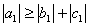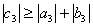16. Gauss Jacobi Method: If the given system of equation is diagonally dominant then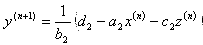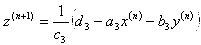17. Gauss Seidel Method: If the given system of equation is diagonally dominant then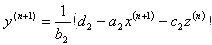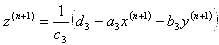18. Sufficient condition for iterative methods (Gauss Seidel Method & Gauss Jacobi Method) to convergence is the coefficient matrix should be diagonally dominant.

19. The iteration method is a self correcting method since the round off error is smaller.

20. Why Gauss Seidel iteration is a method of successive corrections?

Ans: Because we replace approximations by corresponding new ones as soon the latter have been computed.

21. Compare Gauss Elimination Method and Gauss Jordan Method
 Gauss Elimination Method Gauss Jordan Method 1. Direct Method 2. Coefficient matrix is transformed into upper triangular matrix. 3. We obtain the solution by back substitution method. 1. Direct Method 2. Coefficient matrix is transformed into diagonal matrix. 3. No need of back substitution method. Since finally this system of equation each has only one unknown.

22. Inverse of a Matrix: Let A be an nn nonsingular matrix. If X is the inverse of the matrix A then AX = I (i.e.) X = I A-1. We start with augmented matrix of A with identity matrix I of the same order and convert A into the required form (i.e.) identity then the inverse is formed. [A / I ]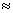[I / A-1].

23. Compare Gauss Elimination Method and Gauss Seidel Method.
 Gauss Elimination Method Gauss Seidel Method 1. Direct Method 2. It has the advantage that it is finite and works in theory for any non-singular set of equation. 3. We obtain exact value. 1. Indirect Method 2. It converges only for diagonally dominant. 3. Approximate value which is self correct method.

24. Compare Gauss Jacobi and Gauss Seidel Methods.
 Gauss Jacobi Method Gauss Seidel Method 1. Indirect Method 2. Convergence rate is slow. 3. Condition for convergence is diagonally dominant. 1. Indirect Method 2. The rate of convergence of this method is roughly twice that of Jacobi. 3. Condition for convergence is diagonally dominant.

25. Why Gauss Seidel method is better method than Jacobi’s method?

Ans: Since the current value of the unknowns at each stage of iteration are used in proceeding to the next stage of iteration, the convergence in Gauss Seidel method will be more rapid than in Gauss Jacobi method.

#### 1 comment:

1.Nice Effort, Well Done - From Ijaz Talib (Assistant Professor of Physics, Govt. Graduate College, Toba Tek Singh, Punjab, Pakistan)